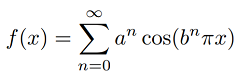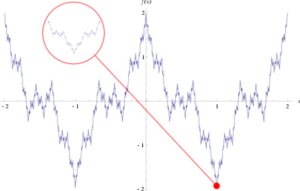# Weierstrass Function

The term Weierstrass function may refer to either

1. A specific continuous function without a defined derivative, or
2. A special function of a complex variable.

## 1. “Nowhere Differentiable” Weierstrass Function

Weierstrass Functions are famous because they are continuous everywhere, yet nowhere differentiable. In general, they are extremely “bumpy”, which leads to problems with finding derivatives at any point.

In notation, the Weierstrass function (Weierstrass, 1872 as cited in Nelson) is defined as:

“Let a ∈ (0, 1) and let b be an odd integer such that ab < 1 + 3π/2. Then the
seriesconverges uniformly on ℝ and defines a continuous but nowhere differentiable function.”

A and B can be any numbers as long as:

• B is between 0 and 1, and
• A * B is larger than 1 + (3 * π/2), which is roughly equivalent to 5.71.

For example A = 12, B = 0.75.

## Graph of the Weierstrass FunctionPlot of Weierstrass function over the interval [−2, 2]. Like some fractals, the function exhibits self-similarity: every zoom (red circle) is similar to the plot as a whole.
Theoretically, you could zoom in forever, and the function would never smooth out enough for the slope of the curve to be calculated.

## 2. Weierstrass Special Function

The Weierstrass Elliptic Function is found in complex analysis, and is a subtype of Elliptic functions, which are classified as either Jacobi or Weierstrass. The difference between the two is that the Weierstrass type has a second order pole at z = 0.

Some variants of the Weierstrass Elliptic Function include the Weierstrass sigma, zeta, eta and ℘-Function (also called the Weierstrass sigma function). The variants are closely related to each other, in the same way that sine function, cotangent function, and squared cosecant functions are related: the logarithmic derivative of the sine function is the cotangent function, whose derivative is the negative squared cosecant function.

## References

Falconer, K. Fractal Geometry: Mathematical Foundations and Applications. New York: Wiley, 1990.
Hairer, E. and Wanner, G. Analysis by Its History. New York: Springer-Verlag, 1996.
Hardy, G. H. “Weierstrass’s Non-Differentiable Function.” Trans. Amer. Math. Soc. 17, 301-325, 1916.
Havil, J. “Weierstrass Function.” §D.2, Appendix D, in Gamma: Exploring Euler’s Constant. Princeton, NJ: Princeton University Press, pp. 230-231, 2003
Nelson, B, The Weierstrass Function. Retrieved December 31, 2019 from: https://math.berkeley.edu/~brent/files/104_weierstrass.pdf
Pickover, C. A. Keys to Infinity. New York: Wiley, p. 190, 1995.
Salzer, H. E. and Levine, N. “Table of a Weierstrass Continuous Non-Differentiable Function.” Math. Comput. 15, 120-130, 1961.
Singh, A. N. The Theory and Construction of Non-Differentiable Functions. Lucknow: Newul Kishore Press, 1935.

CITE THIS AS:
Stephanie Glen. "Weierstrass Function" From StatisticsHowTo.com: Elementary Statistics for the rest of us! https://www.statisticshowto.com/weierstrass-function/